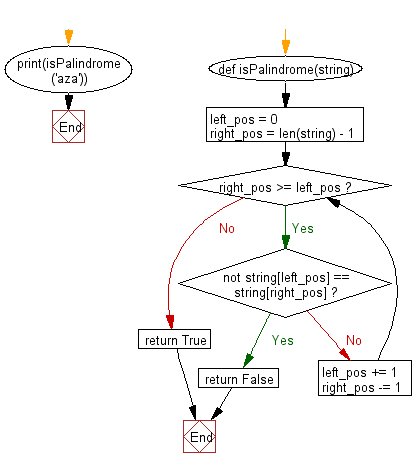﻿ Python Exercise: Checks whether a passed string is palindrome or not - w3resource# Python Exercise: Checks whether a passed string is palindrome or not

## Python Functions: Exercise-12 with Solution

Write a Python function that checks whether a passed string is palindrome or not.

Note: A palindrome is a word, phrase, or sequence that reads the same backward as forward, e.g., madam or nurses run.

Sample Solution:-

Python Code:

``````def isPalindrome(string):
left_pos = 0
right_pos = len(string) - 1

while right_pos >= left_pos:
if not string[left_pos] == string[right_pos]:
return False
left_pos += 1
right_pos -= 1
return True
print(isPalindrome('aza'))
```
```

Sample Output:

```True
```

Pictorial presentation:Flowchart:## Visualize Python code execution:

The following tool visualize what the computer is doing step-by-step as it executes the said program:

Python Code Editor:

Have another way to solve this solution? Contribute your code (and comments) through Disqus.

What is the difficulty level of this exercise?

Test your Programming skills with w3resource's quiz.

﻿

## Python: Tips of the Day

Decapitalizes the first letter of a string:

Example:

```def tips_decapitalize(s, upper_rest=False):
return s[:1].lower() + (s[1:].upper() if upper_rest else s[1:])
print(tips_decapitalize('PythonTips'))
print(tips_decapitalize('PythonTips', True))
```

Output:

```pythonTips
pYTHONTIPS
```